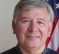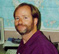## Multiplying the input by the frequency responseStarted by 6 years ago12 replieslatest reply 6 years ago1133 views

Hello All,

Merry Christmas and happy new year to all of you.

Would really appreciate it if someone one can give me a helping hand (advice) with this problem. I have hit a brick wall with it.

If we have a simple 1st order FIR low pass filter: H(e^jw)= 0.5(1+e^-jw)

The following input is applied to the low pass filter: x(nT) = 3*cos((20*pi )/40)

The output in time domain can be determined by substituting 'w' the digital frequency of the input signal into the the frequency response. Determine the corresponding magnitude and phase response .

As the frequency is 10Hz and we are sampling at 40Hz, that would make 10Hz, the -3db point. The magnitude is 0.707 and phase is -45 degrees. The magnitude is multiplied by the magnitude of the input signal and the phase subtracted by the phase of the input signal which is 0, in this case i.e.

y[n] = (0.707)*3*cos(((20*pi )/40-45 degrees))

My question is that I don't know how to prove this. I thought I could determine the impulse response from the frequency response. If the Frequency response is: H(e^jw)= 0.5(1+e^-jw) , the impulse response is h[n] ={0.5, 0.5}, starting at n=0.

then y[n]=h[n] * x[n], because signal is periodic circular convolution must be used.

Is this the right approach as I get stuck here, please let me know if I have not explained question properley.

Jag.

[ - ]I'm not sure that I have all the points clear but here goes:

1) The steady state output in time domain can be determined by substituting 'w' the digital frequency of the input signal into the the frequency response.

2) Determine the corresponding magnitude and phase response .

??An assertion followed by an objective.  mmmm OK.  Sounds like a homework assignment. Why bother to say: "DIGITAL frequency"?  Does this imply discrete frequency?

How to prove?  I'm not sure how "determining the impulse response from the frequency response" factors into this objective - maybe....

And, are you sure that you aren't needing to determine the unit sample response - as that would fit the discrete frequency representation.

All of the notation, except H(e^-jw) is in discrete terms so it's a bit troublesome to know exactly what you have in mind in going from a continuous infinite frequency function to a discrete time function.  That said, it may well be done this way (somehow) but it's unusual so it raises unnecessary questions.  This may be a reason for "getting stuck".

[ - ]Thank you Fred for your reply, much appreciated. I am getting a little confused with it. As you say its unconventional and as such I have not been able to find much help on internet. The frequency response shown was also discrete, 'w' is (2*pi*f)/Fs. I was trying to confirm my answer which I simply got by subbing 'w' into the frequency response.

You have given me a lot to think about.

[ - ]I would prefer to say it this way:

There is temporal extent of (N+1)*T where T is the sample interval in time and N are the number of samples in time.  The "+1" comes because we assume the entire record of samples repeats at time = (N+1)*T and the sample at that time is not in the record of N (repeating) samples and is equal to the sample value at time=0.

The temporal sample rate Fs = 1/T.

The frequency extent is Fs and the frequency sample interval is Fs/N = 1/NT.
There are N frequency points with data (complex) and the sample at Fs is assumed to be a repeat of the sample at zero - that's where the period of the frequency values starts repeating.

You may note that the frequency resolution (sample interval) is the reciprocal of the temporal record window (really the temporal period length) so (N+1)T
AND
the time resolution (sample interval) is the reciprocal of the frequency record window Fs (really the frequency period length) so 1/Fs.

Then, in order to plug in "w", it would better be stated as plugging in:

k*2pi*Fs/N where k is less than 0.5 and represents the index of the frequency you want.
Negative frequency components are similarly located below Fs and above Fs/2.

Until you get used to it, it may be a bit confusing to deal with negative frequencies arranged in the arrays this way.  I find there's a lot of good learning to be had by using purely real and even functions and purely real and odd functions and then working with FFT, IFFT and plots.

Example:
100 samples in time with interval 1 second.
Temporal range of those samples is N=99 seconds from start to finish.
N+1=100 so the temporal period is 100 seconds.
Frequency resolution or sample interval is 1/100Hz which is the reciprocal of 100 seconds .
Frequency extent Fs is 1Hz which is the reciprocal of the 1 second temporal sample rate.

[ - ]Hi Jag,

You have the general understanding of the problem, but there are several math and conceptual errors.

You start off with the frequency response of a continuous signal: H(e^jw)= 0.5(1+e^-jw)but then switch to discrete signals:  x(nT) = 3*cos((20*pi )/40).  So it seems to me you are mixing up the Laplace and z-transform math.

Your equation for a time domain cosine wave is missing the independent variable. You write:  x(nT) = 3*cos((20*pi )/40),  which is read:  "x as a function of n and T", but you equation doesn't use the variables n or T.

You are mixing up radians and degrees: y[n] = (0.707)*3*cos(((20*pi )/40-45 degrees)).  20*pi/40 is in radians.  45 is in degrees.

You write: "because signal is periodic circular convolution must be used."  That's not correct, this is not a case of circular convolution.

You overall method of proving a simple example is good.  If the impulse response is given by:  h[n] ={0.5, 0.5},  you should write the time domain equation as a sum of two Cosine waves, one that corresponds to the first 0.5, and the other that corresponds to the second 0.5.  The second Cosine wave is shifted by one sample in the time domain.  Then show that the sum of these two Cosine waves is another Cosine wave with amplitude 0.707 with a 45 degree phase shift.

Hope this helps... you have a lot of areas that need to be cleaned up.

Regards,

Steve

[ - ]Hello Steve,

Thanks for your reply, I had a bash at a solution by taking the Z transform of the input, then multiplying that by the transfer function and taking the inverse Z Transform using PFE method. Not sure I have done it correctly, I have attached screen shots.

I am a little confused about the discrete time, I know the impulse response and input have to be convolved but dont know why you are asking me to write  2 cosines to match the impulse response samples. I thought they would be convolved by the samples for a single period of the cosine wave. I apologise If I am missing some basic knowledge.

Jag.Question.png

[ - ]Looks much better!

Convolution is a single well-defined mathematical operation, taking two signals and creating a third.  However, there are a number of different ways that convolution can be viewed or interpreted.  Here's one:

Say you have an arbitrary input signal, x[k], and an impulse response that consists of h = 0.8, h = 0.5, and h = 0.3.   Each of the three points in the impulse response will result  in a copy of x[k] appearing in the output signal, y[k], with an appropriate scaling and shift.  In this particular example:

y[k] = 0.8*x[k] + 0.5*x(k-1] + 0.3*x(k-2]

So if you have a very simple impulse response, the easiest way to calculate the convolution is to just write the above equation.  For your input signal being a sinusoid, and the impulse response of [0.5,0.5], this is the sum of two sinusoids shifted by one sample.

[ - ]Thank you Steve :)

[ - ]A picture is worth thousand words

run the following code in Matlab, here I check your filter system in two ways and compare, I then apply an input in three ways and compare...

you will notice that you have attempted the TF approach which is the most difficult path that I didn't bother to complete.

_____________________________________________________

%filter system

w = -pi:.01:pi;          %discrete frequency grid

H1 = 0.5*(1+exp(-j*w));  %TF

H2 = fftshift(fft([.5 .5],length(w)));%using fft

figure; plot(abs(H1),'-');hold

plot(abs(H2),'r--');

%given input/output

x = cos((0:length(w)-1)*2*pi*.2);%f = .2

y1 = filter([.5 .5],1,x); %get output using convolution in time domain

y2 = fft(x,length(w)).*fft([.5 .5],length(w)); %multiplication in freq domain

y2 = ifft(y2); %and back to time domain

figure;plot(abs(y1),'-');hold

plot(abs(y2),'--r');

%get output using TF with w = that of input, Not completed

y3 = 0.5*(1+exp(-j*.2*(2*pi)));

y3 = ifft(y3,length(w));  %wrong location in ifft frame

[ - ]Thank you so much Kaz

[ - ]If you are saying I got two coeffs 0.5, 0.5 for fir i.e. Hz = 0.5 + 0.5z-1

I get the frequency response from z transfer function => H(e^jw)= 0.5(1+e^-jw)

then I want to get those coeffs back from the frequency response

you can do ifft as follows:

w = -pi:.1:pi;

H = 0.5*(1+exp(-j*w));

y = fftshift(ifft(H));

select the centre two y values which should be 0.5,0.5

[ - ][ - ]